## Runge Kutta-3

Suppose we have ODE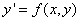then

Formula :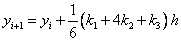Where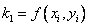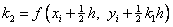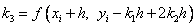Error term :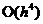, correct up to the third order term in Taylor series expansion.

The Taylor series expansion is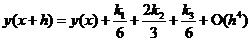Example:, set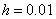, with initial condition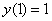. The few first results and the graph of solution are given below.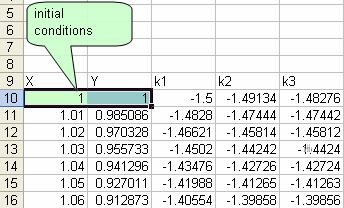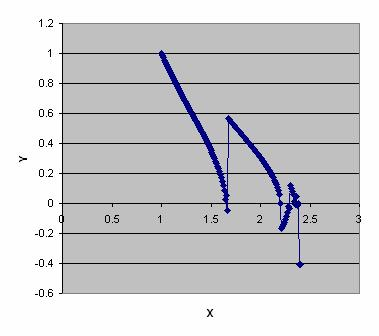Note that after, the solution is not correct. See Comparison .

Preferable reference for this tutorial is

Teknomo, Kardi (2015) Solving Ordinary Differential Equation (ODE). https:\\people.revoledu.com\kardi\tutorial\ODE\